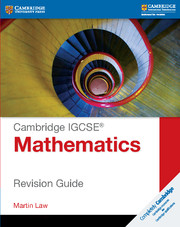× This is a Sterling guide price. You will be invoiced in the appropriate currency for your country.
• ISBN:9781107611955
• Format:Paperback
• Subject(s):Mathematics
• Qualification:Cambridge IGCSE
• Author(s):Martin Law
• Available from: March 2016

Cambridge IGCSE Mathematics Revision Guide provides a thorough resource to help students prepare and practice the skills required for the 0580 examination.

× This is a Sterling guide price. You will be invoiced in the appropriate currency for your country.

Send a Query

formRow
×
• DESCRIPTION

This Revision Guide provides a thorough resource to help students prepare and practice the skills required for the Cambridge IGCSE Mathematics 0580 syllabus. Written by an experienced mathematics teacher, Martin Law, with over 20 years of teaching experience, this resource can be used as an ongoing reference book or as an aid for final exam preparation. The Cambridge IGCSE Mathematics: Revision Guide directly supports the latest Cambridge International Examinations syllabus with clear explanations of the mathematical techniques that students need to know, as well as exam-style questions with each step fully explained to help reinforce understanding. Each chapter contains revision learning objectives at the start and key points for effective preparation.

• Contents
• FEATURES
• Clear objectives at the start of each chapter to help students plan revision.

• Concise explanations of the essential content to help reinforce understanding.

• Exam-style questions to test knowledge.

• CONTENTS
• Revision checklist
• Formulae
• Introduction
• 1. Number, set notation and language
• 2. Squares, roots and cubes, directed numbers, fractions, decimals and percentages
• 3. Ordering, standard form, four rules and estimation
• 4. Limits of accuracy
• 5. Ratio, proportion and rate
• 6. Percentages
• 7. Use of calculator, measures and time
• 8. Money, personal and household finance
• 9. Graphs in practical situations
• 10. Graphs of functions
• 11. Straight line graphs
• 12. Algebraic representation and formulae
• 13. Algebraic manipulation
• 14. Functions
• 15. Indices
• 16. Solution of equations and inequalities
• 17. Linear programming
• 18. Geometric terms and relationships
• 19. Geometrical constructions
• 20. Symmetry
• 21. Angle properties
• 22. Locus
• 23. Mensuration
• 24. Trigonometry
• 25. Statistics
• 26. Probability
• 27. Vectors
• 28. Matrices
• 29. Transformations
• Core practice papers
• Extended practice papers

Not already registered? Create an account now. ×

Other Cambridge websitesNeed a local sales consultant?